210610 Epaper

 A Section B SectionC Section D Section210609 Epaper

 A Section B SectionC Section D Section210608 Epaper

 A Section B SectionC Section D Section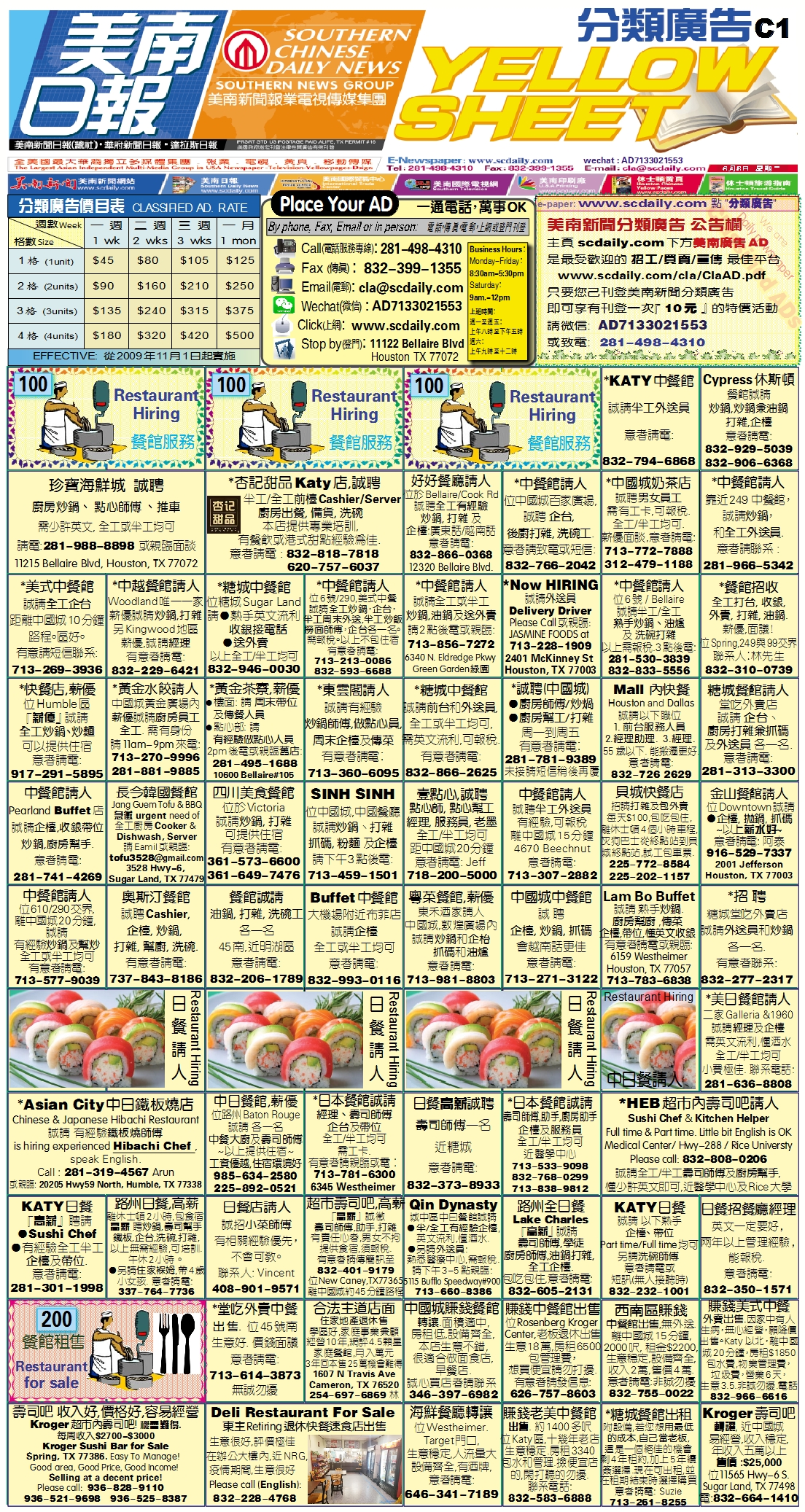210607 Epaper

 A Section B SectionC Section D Section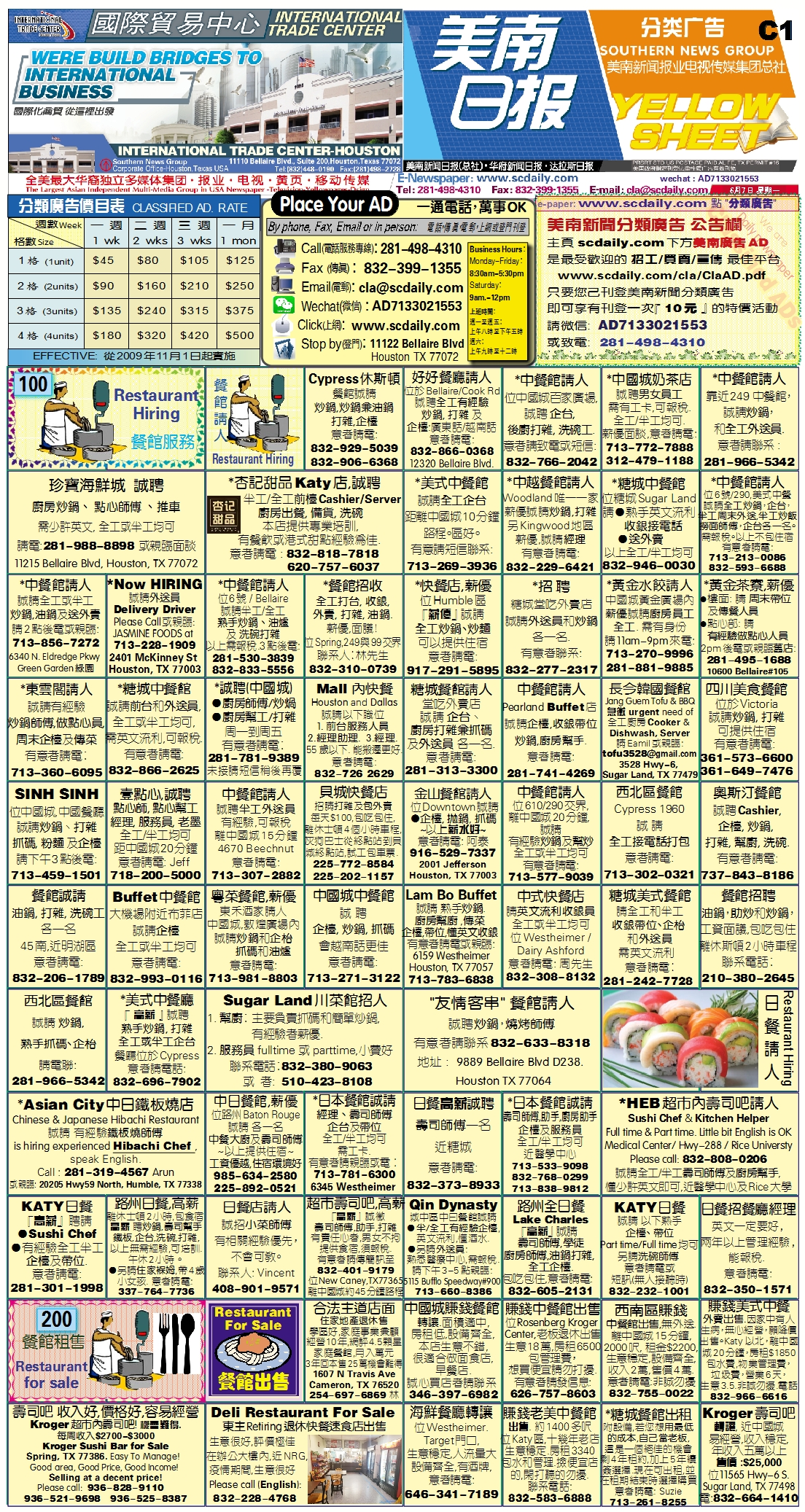210606 Epaper

 A Section B SectionC Section D Section210605 Epaper

 A Section B SectionC Section D Section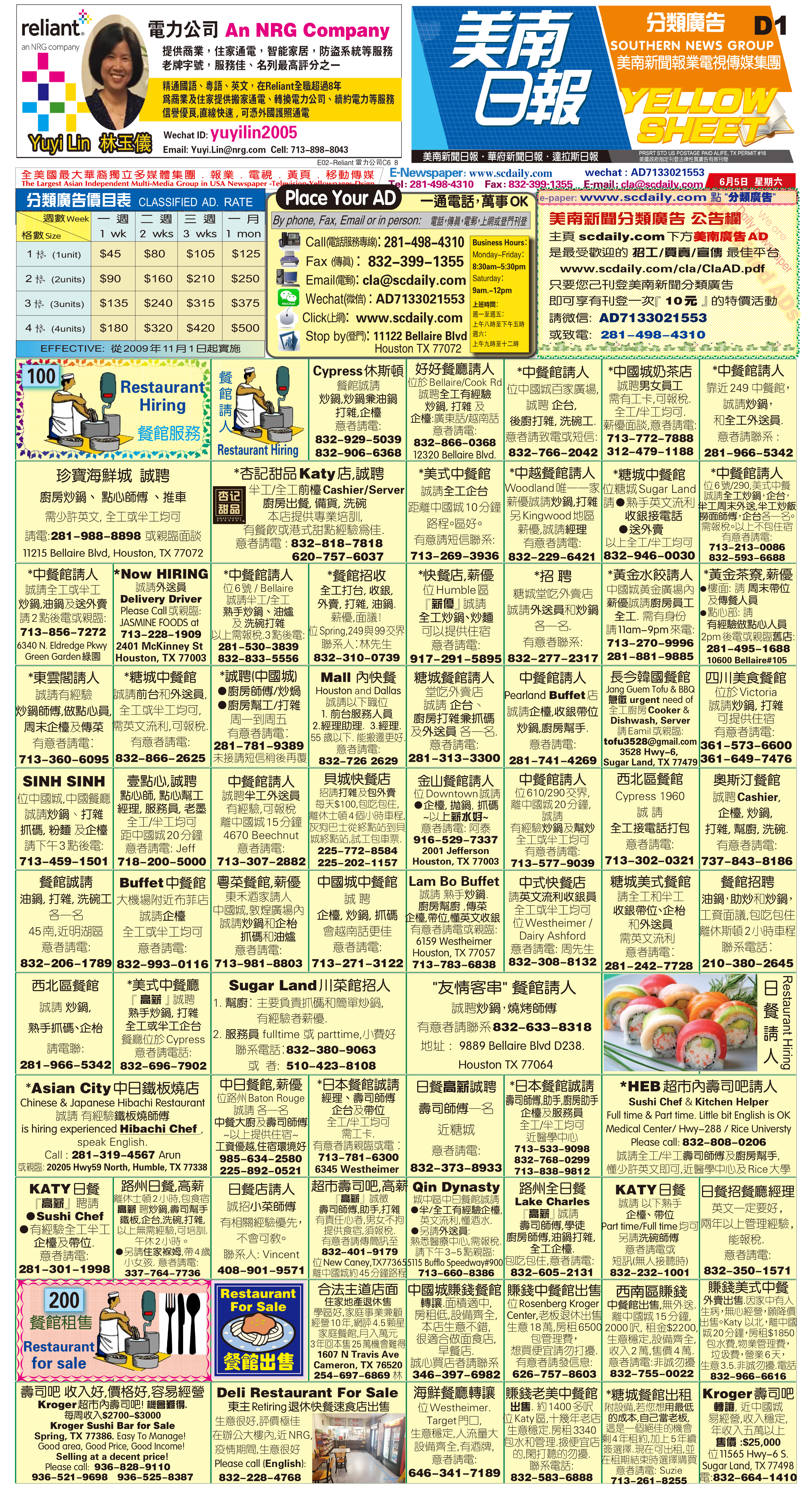210604 Epaper

 A Section B SectionC Section D Section210603 Epaper

 A Section B SectionC Section D Section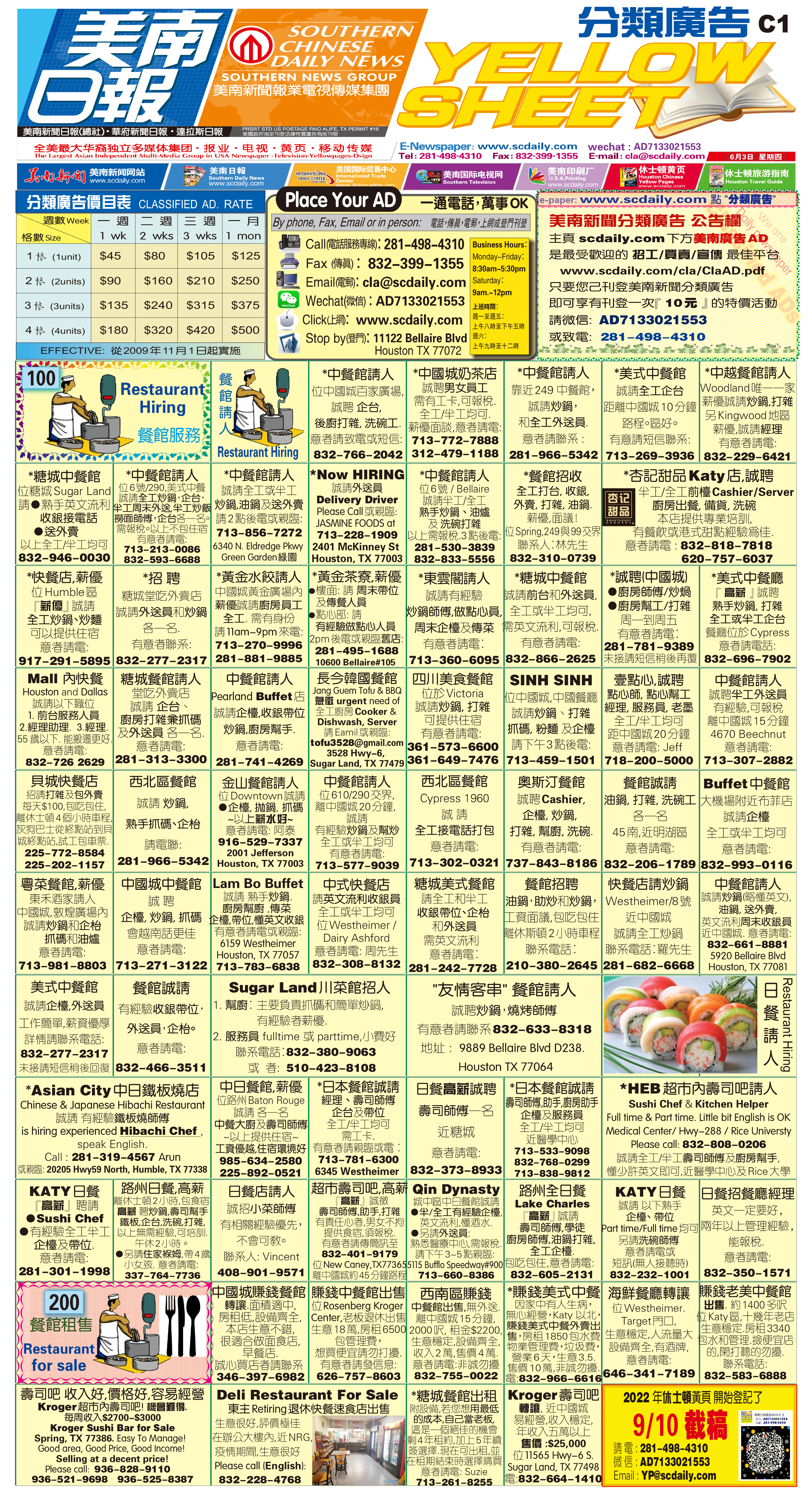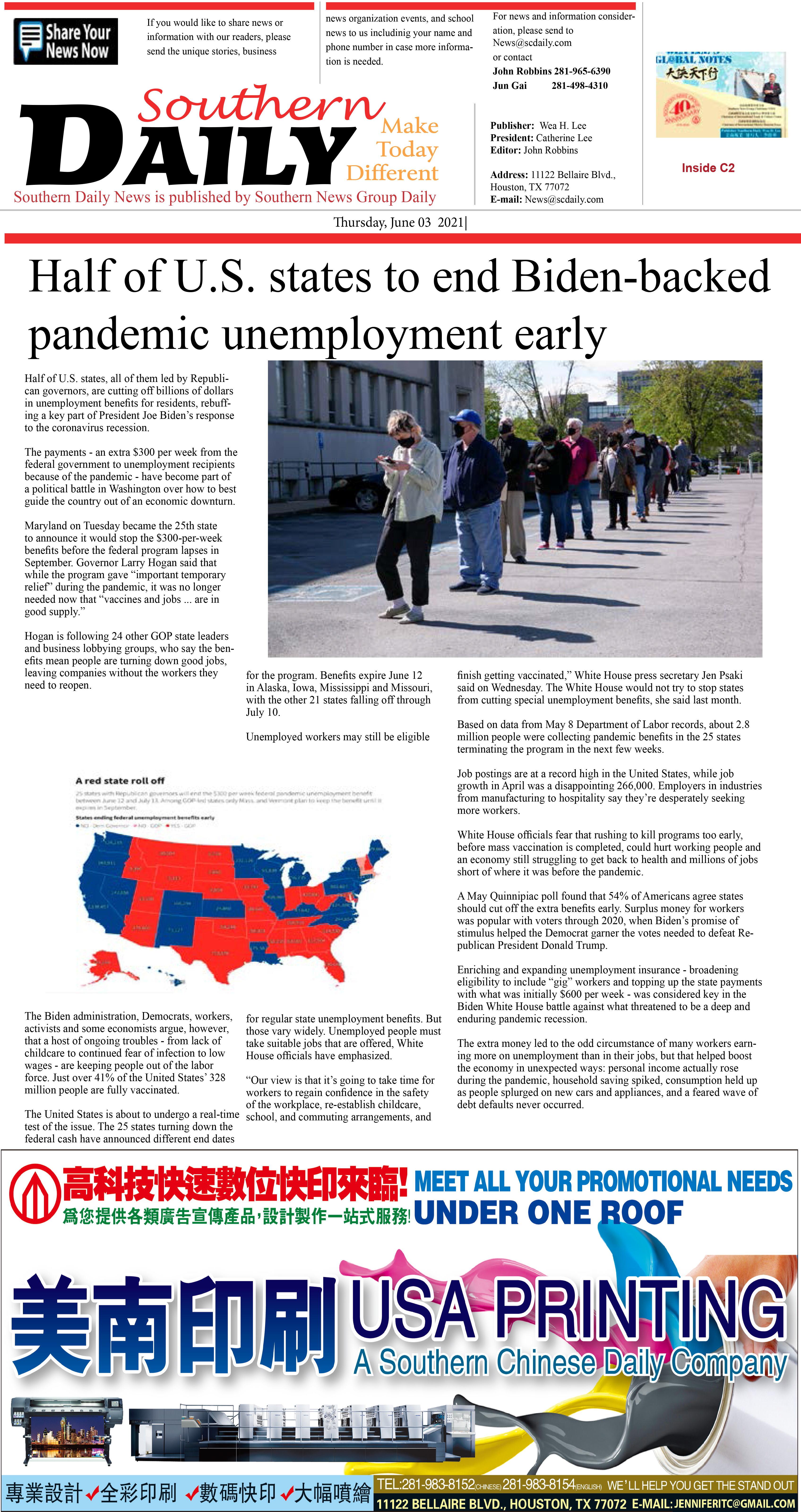210601 Epaper

 A Section B SectionC Section D Section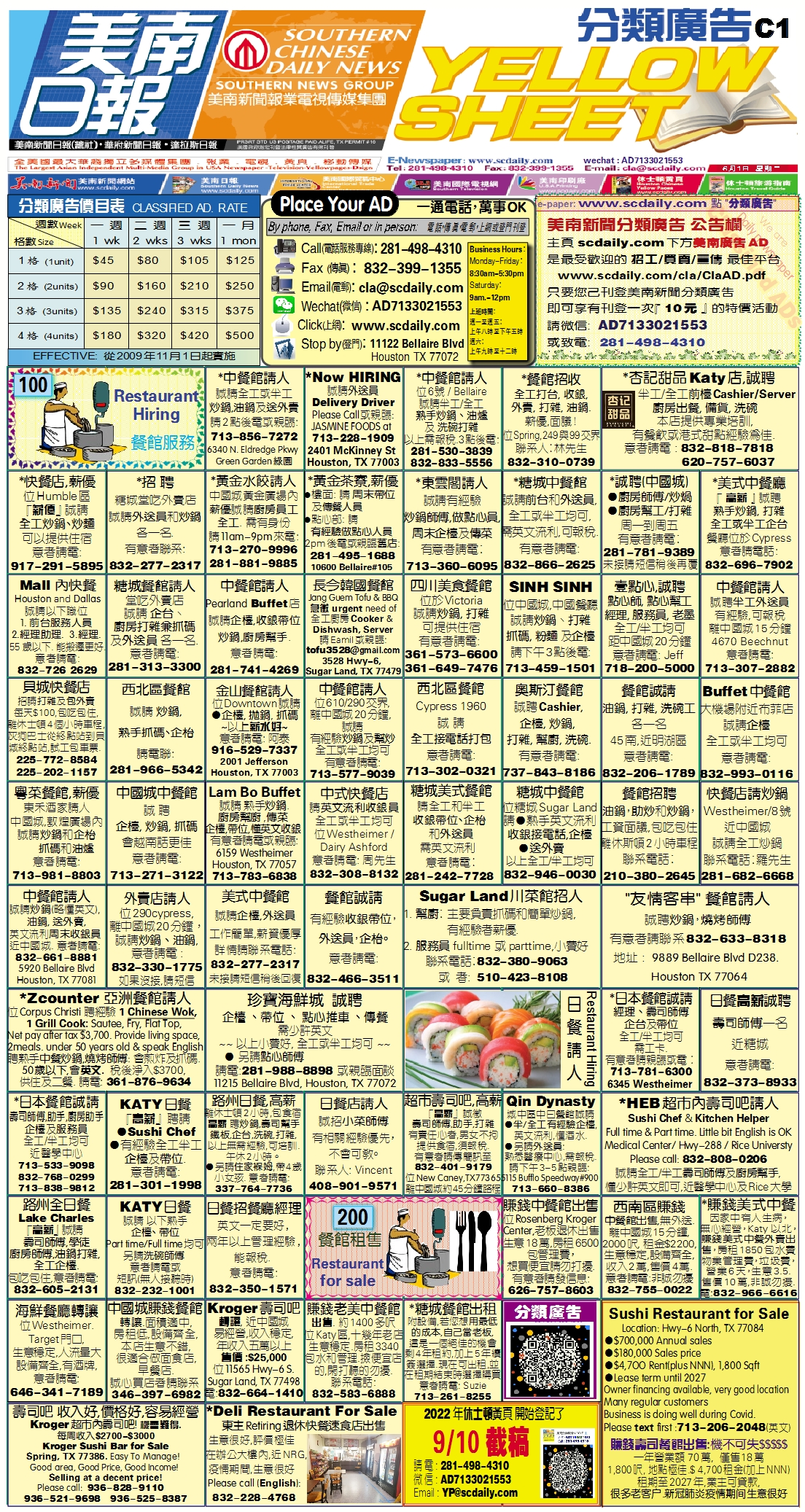210602 Epaper

 A Section B SectionC Section D Section210531 Epaper

 A Section B SectionC Section D Section